## Calculate Thanksgiving Date

This post will guide you how to calculate the thanksgiving data based on a specific year date using a formula or a User Defined Function with VBA Code in excel 2013/2016/2019/365. How do I get the thanksgiving date based on a specific years in excel.

## 1. Calculate the US Thanksgiving Date using a Formula

You can use a simple arithmetic to calculate the possible date range for the US Thanksgiving, it falls on the fourth Thursday in November. And you can create a formula based on the DATE function and the WEEKDAY function.

To Calculate the US Thanksgiving date, you can write down the following formula:

``=DATE(B2,11,29)-WEEKDAY(DATE(B2,11,3))``

Or

``=DATE(C2,11,29)-WEEKDAY(DATE(C2,11,24))``

You just need to type this formula in the formula box of cell C2, and press Enter in your keyboard. Then drag the AutoFill Handler over other cell to apply this formula.

## 2. Calculate Thanksgiving Date using a User Defined Function with VBA Code

If you want to calculate the Thanksgiving date using a user-defined function in Excel, you can follow these steps:

Step1: Press ALT + F11 to open the Visual Basic Editor.

Step2: In the Visual Basic Editor, click Insert > Module.

Step3: In the Module window, paste the following code:

```Function ThanksgivingDate_Excelhow(ByVal Year As Integer) As Date
ThanksgivingDate_Excelhow = DateSerial(Year, 11, 29) - Weekday(DateSerial(Year, 11, 3))
End Function```

Step4: Save the module and close the Visual Basic Editor.

Step5: enter the year you want to calculate the Thanksgiving date for in a cell, for example, cell A2.  In another cell, enter the formula:

``=ThanksgivingDate_Excelhow(B2)``

Step6: press Enter key, the cell will now display the Thanksgiving date for the year you entered in cell A2.

## 3. Video: Calculate Thanksgiving Date in Excel

This video shows how to calculate the Thanksgiving date in Excel using both the DATE function and a user-defined function with VBA code.

## 4. Related Functions

• Excel DATE function
The Excel DATE function returns the serial number for a date.The syntax of the DATE function is as below:= DATE (year, month, day)…
• Excel WEEKDAY function
The Excel WEEKDAY function returns a integer value representing the day fo the week for a given Excel date and the value is range from 1 to 7.The syntax of the WEEKDAY function is as below:=WEEKDAY (serial_number,[return_type])…

## How to Count Dates by Day of Week in Excel

This post will guide you how to count days of week in the range of dates using a formula in Excel 2013/2016 or Excel office 365. How do I count dates by day of week in Excel.

## Count Dates by Day of Week Through Formula

Sometimes, you may be want to count days of week in a given date range or between two dates. And you need to build a complex formula to work with your data. To count dates by weekday(such as: Mondays, Tuesdays, etc), and you can use the SUMPRODUCT function in combination with the WEEKDAY function to create a formula in Excel.

For example, your date range is B1:B6, and you want to count dates by weekday, just using the following formula:

=SUMPRODUCT(–(WEEKDAY(\$B\$1:\$B\$6)=D1))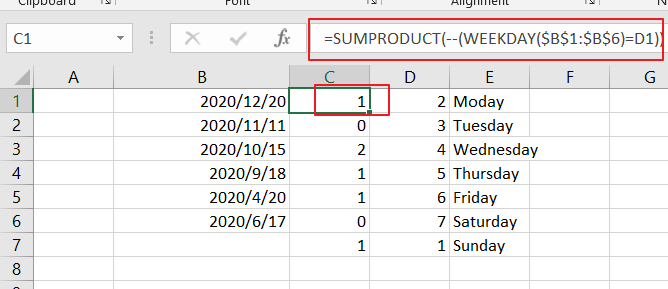LETS SEE THAT HOW THIS FORMULA WORKS:

=WEEKDAY(B1：B6)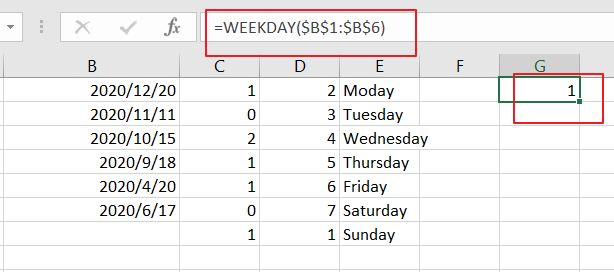The formula WEEKDAY returns a number between 1 and 7 based on a particular day of the week. Such as: Sunday is 1, Monday is 2, Thuesday is 3, Wednesday is 4, Thursday is 5, Friday is 6 and Saturday is 7.

This formula returns an array like this:

{1;4;5;6;2;4}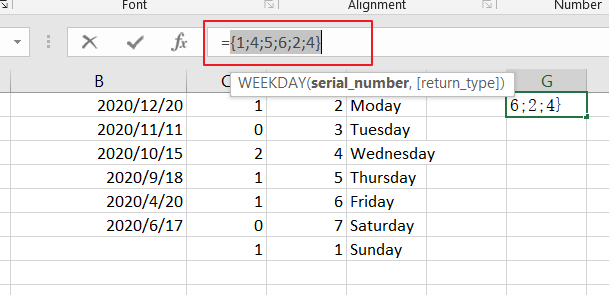=WEEKDAY(\$B\$1:\$B\$6)=1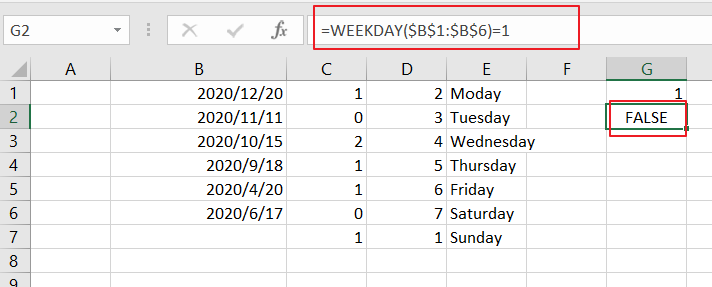Then the numbers returned by WEEKDAY formula are compared to the given day value of weekday, returns another array result like this:

{TRUE;FALSE;FALSE;FALSE;FALSE;FALSE}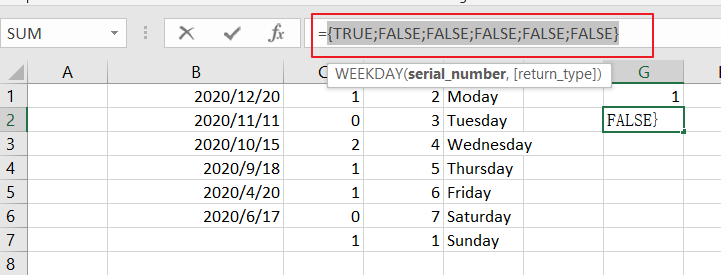=–(WEEKDAY(\$B\$1:\$B\$6)=1)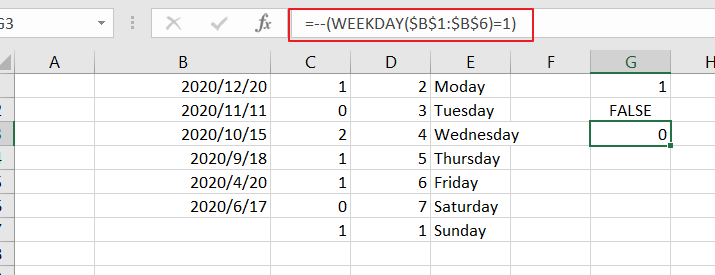You still need to convert the TRUE or FALSE values to 1 and 0 values using the double negative operator. And returns the below array result:

{1;0;0;0;0;0}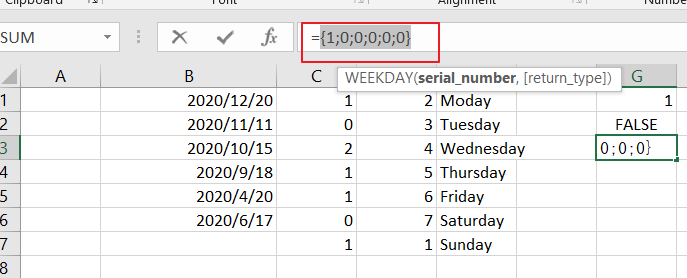The SUMPRODUCT function just sums all values of an array.

### Related Functions

• Excel SUMPRODUCT function
The Excel SUMPRODUCT function multiplies corresponding components in the given one or more arrays or ranges, and returns the sum of those products. The syntax of the SUMPRODUCT function is as below:= SUMPRODUCT (array1,[array2],…)…
• Excel WEEKDAY function
The Excel WEEKDAY function returns a integer value representing the day fo the week for a given Excel date and the value is range from 1 to 7.The syntax of the WEEKDAY function is as below:=WEEKDAY (serial_number,[return_type])…

## How to Sum Data by Weekday with Different Formulas/Functions in Excel

To generate a business summary report for further investigation, we can sum income or profits for a date like Monday, Tuesday …etc. to check on which date we have the top income in a week, then we can estimate the market trend. Actually, sum data by weekday is a common operation in our daily life, when generating report, we often select one or more weekdays as a period to sum data. So, it is necessary for us to have the knowledge of sum data by weekday in excel. Actually, excel built-in functions SUMIFS and SUMPRODUCT can help us resolve this issue properly.

This article will show you ‘Sum by Weekday’ in two different ways via excel WEEKDAY, SUMIFS and SUMPRODUCT functions with simple examples, descriptions, screenshots and explanations, and we will let you know how these formulas work with these functions. Besides, there are some differences in the usage of the two functions SUMIFS and SUMPRODUCT even though they are both used for sum data. Read the following article, you can learn sum data by formula clearly by yourself, you can select one way you feel easy to understand to achieve your goal, and you can work well with these functions in your daily work in the future.

EXAMPLE: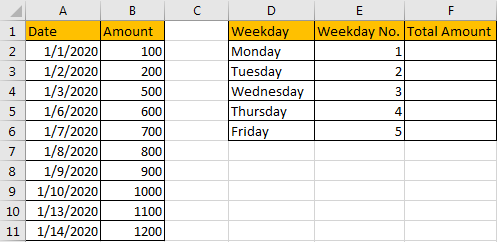## METHOD 1: APPLY SUMIFS FUNCTION TO SUM DATA BY WEEKDAY

As we can see that there is a column in the right table called ‘Weekday No.’, actually each value reflects a weekday accordingly, for example 1 reflects Monday, 2 reflects Tuesday… and 5 reflects Friday. So, we can also create a helper column called ‘Weekday No.’ in the left table, then we can use SUMIFS function contains ‘Weekday No.’ as criteria range to sum data by weekday properly. Refer to this method, the first step we need to get weekday numbers for all entered data in ‘Date’ list. So, create a helper column, enter the formula =WEEKDAY(A2,2) in the blank cell, see screenshot below.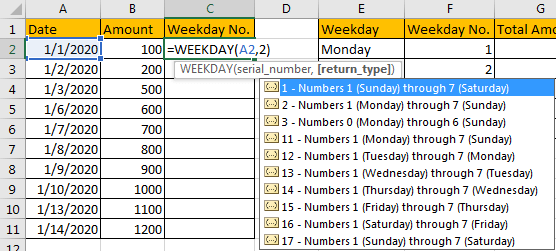As generally if you omit ‘return type’ in WEEKDAY function, Sunday will be assigned with weekday number 1 by default, so the weekday numbers are inconsistent in two ‘Weekday No.’ columns. So here we enter ‘2’ as return type, then Monday will be assigned with weekday number 1.

After clicking Enter, the formula returns ‘3’ as result. That means date 1/1/2020 is Wednesday.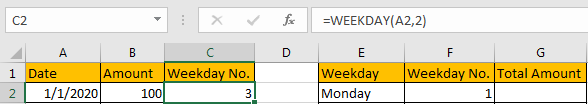Drag down the cell to copy the formula. Then we can get all weekday numbers for entered dates.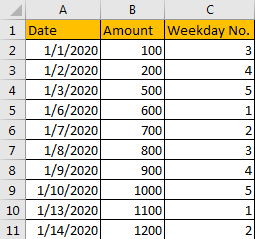Now, Weekday No. in C column can be seen a criteria range for SUMIFS function, and each number in ‘Weekday No.’ in F column can be seen as criteria of it. Now, we can apply SUMIFS function in this instance to sum data per weekday.

In G1 enter the formula =SUMIFS(\$B\$2:\$B\$11,\$C\$2:\$C\$11,F2). After clicking Enter, we can get correct total amount 1700, that means the formula works correctly.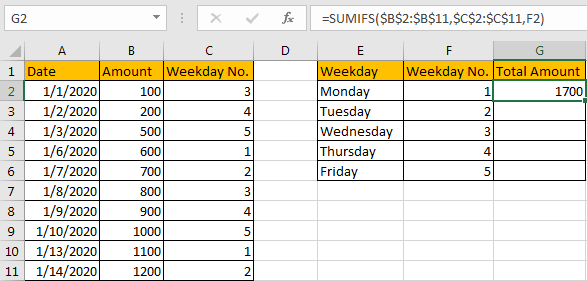HOW THIS FORMULA WORKS:

This formula contains two functions, we explain the formula from inside to outside. Firstly, we apply WEEKDAY function in this instance. WEEKDAY function can return the day of a week, it returns an integer, and the integer range is 1 to 7, normally 1 represents Sunday, 2 represents Monday etc., and 7 represents Saturday. The syntax is consistent of WEEKDAY(serial_number,[return_type]).

You can enter a date within double quote in the function. For example, enter =WEEKDAY(“10/22/2020”), click Enter, we will get 5. That means 10/22/2020 is Thursday.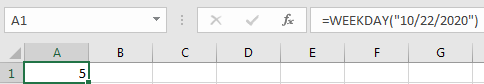But if you want to set 1 to represent Monday, 2 represent Tuesday and 7 represent Sunday respectively, we can add return type 2 into WEEKDAY function. Now 4 is returned as we expect.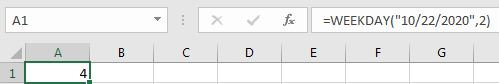We can also select a cell number directly when applying this function. In this instance, we can get correct weekday number after applying the formula.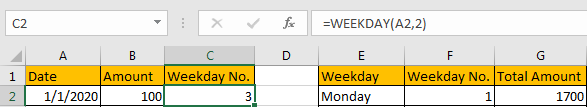Then we apply SUMIFS function =SUMIFS(\$B\$2:\$B\$11,\$C\$2:\$C\$11,F2) to sum data. For SUMIFS function, it sums data with multiple criteria, the syntax has some arguments, see below:

=SUMIFS(sum_range, criteria_range1, criteria1, [criteria_range2, criteria2], …).

\$B\$2:\$B\$11 is the sum range, it provides values to sum, as it is an absolute range in this case, so we add \$ before row and column number to lock the range, so when copying formula to other cells, this range is fixed, user doesn’t need to adjust the sum range any more.

\$C\$2:\$C\$11 is the criteria range, it provides all weekday numbers, it is an absolute range in this case as well, so we add \$ before row and column number.

F2 is the criteria of criteria range \$C\$2:\$C\$11.

We compare each value in criteria range \$C\$2:\$C\$11 with the value in F2, if they are consistent, corresponding amount value in sum range \$B\$2:\$B\$11 will be accumulated. By this way, we get total amount for each weekday.

RESULT:

Let’s check if the formula works well for other weekdays.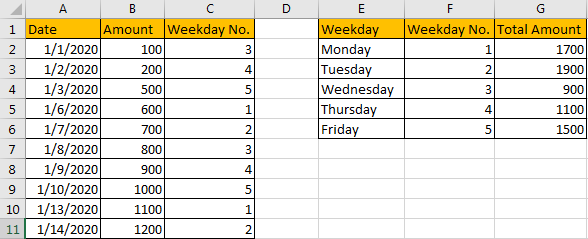## METHOD 2: APPLY SUMPRODUCT FUNCTION TO SUM DATA BY WEEKDAY

The above instance uses a helper column ‘Weekday no.’ to sum data, without helper column SUMIFS function cannot work due to there is no criteria. If we want to sum data by weekday without helper column and just use the given data in tables, how can we do?

Actually, we can through applying another excel function to achieve our requirement.

FORMULA:

In F1 enter the formula =SUMPRODUCT((WEEKDAY(\$A\$2:\$A\$11,2)=E2)*(\$B\$2:\$B\$11)).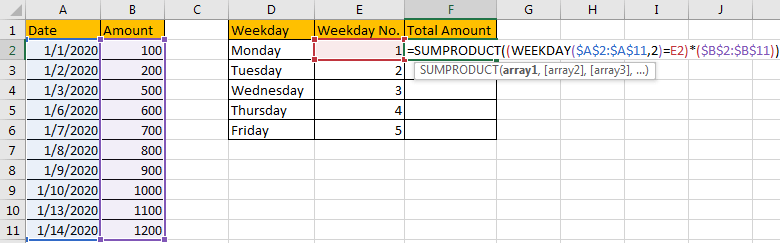Click Enter to check returned value.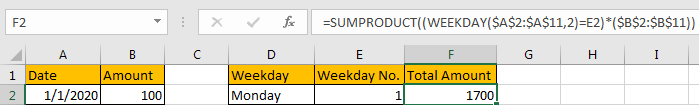Verify that total amount is 1700, it is consistent with the value summed by SUMIFS function in former instance.

HOW THIS FORMULA WORKS:

Unlike SUMIFS function, SUMPRODUCT function syntax has the following arguments:

=SUMPRODUCT(array1, [array2], [array3], …)

In this instance, in the formula SUMPRODUCT((WEEKDAY(\$A\$2:\$A\$11,2)=E2)*(\$B\$2:\$B\$11)), there is only one argument (WEEKDAY(\$A\$2:\$A\$11,2)=E2)*(\$B\$2:\$B\$11). In method #1, we have explained the usage of WEEKDAY, here, as we enter \$A\$2:\$A\$11 as serial number, so WEEKDAY(\$A\$2:\$A\$11,2) will return an array instead of an integer; as return type is ‘2’, so if date is Monday, 1 is returned in array.

In this instance, WEEKDAY(\$A\$2:\$A\$11,2) returns the array {3;4;5;1;2;3;4;5;1;2}.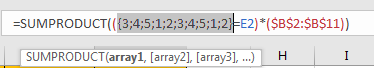(Select WEEKDAY(\$A\$2:\$A\$11,2) in formula bar, and press F9, then returned array will be displayed.)

Just pick up each value from the array list, and compare it with the value in E2 (1 in this case). {3;4;5;1;2;3;4;5;1;2}=1.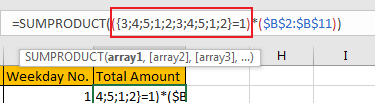After comparing, we can get the result as below:

{FALSE;FALSE;FALSE;TRUE;FALSE;FALSE;FALSE;FALSE;TRUE;FALSE}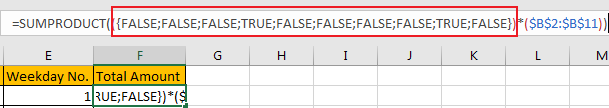As function cannot work with TRUE or FALSE, so they are forced to be converted to numbers. Convert FALSE to 0 and TRUE to 1 for following work. So, the array is updated to {0;0;0;1;0;0;0;0;1;0}.

\$B\$2:\$B\$11 is the sum range {100;200;500;600;700;800;900;1000;1100;1200}.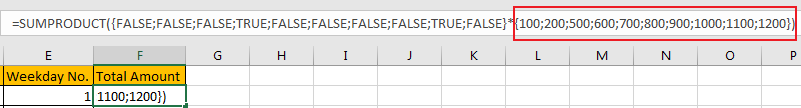So, SUMPRODUCT((WEEKDAY(\$A\$2:\$A\$11,2)=E2)*(\$B\$2:\$B\$11)) can be seen as one array is multiplied  by the values in another array:

{0;0;0;1;0;0;0;0;1;0}*{100;200;500;600;700;800;900;1000;1100;1200}={0;0;0;600;0;0;0;0;1100;0}. Then sum the values in the array, the final result is 1700, see screenshot below.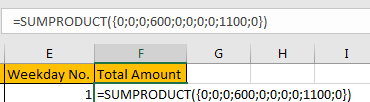RESULT:

Let’s see if this formula works correctly for other weekdays. Obviously, it works well.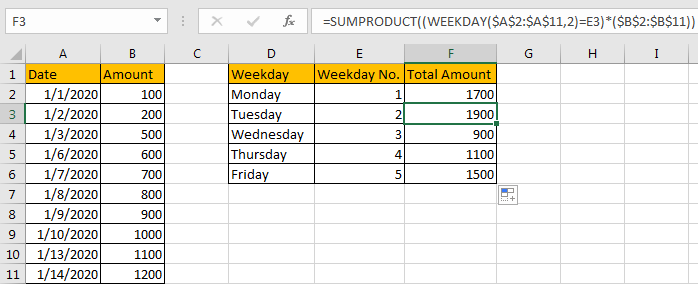NOTES:

If you are confused of range selection in the formula, you can also name range firstly, for example

\$A\$2:\$A\$11=Date;

\$B\$2:\$B\$11=Amount;

To name range, just select the whole range (for example A2:A11, ignore the header), and enter name in name box (for example ‘Date’). Please be aware that there should be NO space in range name.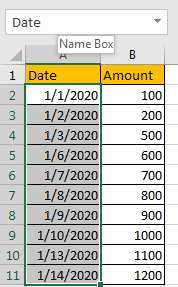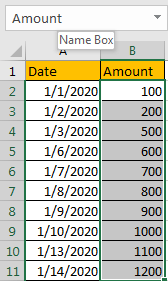Then update formula to =SUMPRODUCT((WEEKDAY(Date,2)=E2)*Amount). It looks clearly now.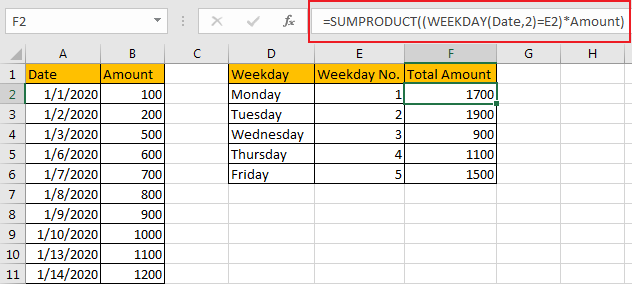### Related Functions

• Excel SUMPRODUCT function
The Excel SUMPRODUCT function multiplies corresponding components in the given one or more arrays or ranges, and returns the sum of those products.The syntax of the SUMPRODUCT function is as below:= SUMPRODUCT (array1,[array2],…)…
• Excel WEEKDAY function
The Excel WEEKDAY function returns a integer value representing the day fo the week for a given Excel date and the value is range from 1 to 7.The syntax of the WEEKDAY function is as below:=WEEKDAY (serial_number,[return_type])…
• Excel SUMIFS Function
The Excel SUMIFS function sum the numbers in the range of cells that meet a single or multiple criteria that you specify. The syntax of the SUMIFS function is as below:=SUMIFS (sum_range, criteria_range1, criteria1, [criteria_range2, criteria2], …)…

## How to Highlight Cell or Row If Date Is In Current Day/Week/Month in Excel

This post will guide you how to highlight cell if date is the current day or is in the current week or month in Excel. How do I highlight row if date is in current week or month with conditional formatting in Excel 2013/2016.

## Highlight Cell if Date is in Current Day/Week/Month

To highlight the cell of current day or the date is in the current week or month, you can do the following steps:

#1 select the range of cells that you want to highlight date.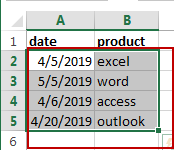#2 go to HOME tab, click Conditional Formatting command under Styles group, and click Highlight Cells Rules menu from the drop down menu list, then select A Date Occurring sub menu. And the A Date Occurring dialog will appear.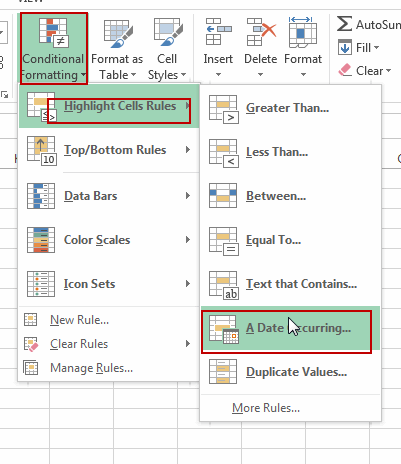#3 if you want to highlight the current day, then select Today option from the first drop down list box in the A Date Occurring dialog box, then choose one color that you want to fill the cell in the second drop down list box. Click Ok button.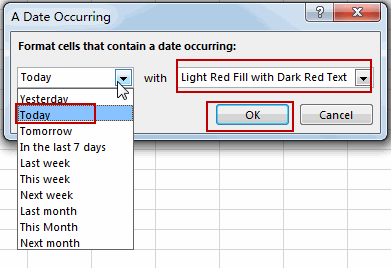Note: if you want to highlight the cell if date is in the current week, just choose This Week option from the first drop down list box. And if you want to highlight the cell if date is in the current month, just choose This Month from the first drop down list box.

#4 you should notice that the current date has been highlighted.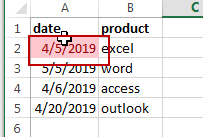## Highlight Row if Date is in Current Day/Week/Month

If you want to highlight the row that If the date in that row is equal to the current day or is in the current week or month, you can do the following steps:

#1 select the entire rows of data that contain date values.#2 go to HOME tab, click Conditional Formatting command under Styles group, Click New Rule from the drop down menu list. And the New Formatting Rule dialog will open.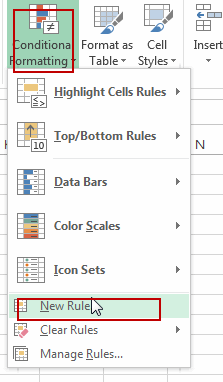#3 click Use a formula to determine which cells to format option in the New Formatting Rule dialog box, type the following formula in the Format values where this formulas is true text box.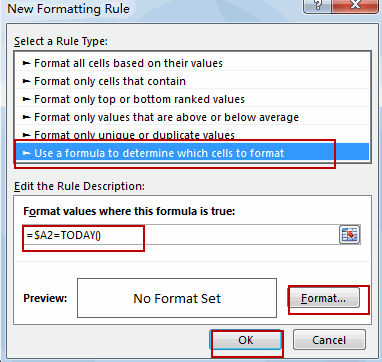To highlight current date, use the following formula:

=\$A2=TODAY()

To highlight row if date is in the current week, use the following formula:

=TODAY()-WEEKDAY(TODAY(), 3)=\$A2-WEEKDAY(\$A2, 3)

To highlight row if date is in the current month, use the following formula:

=TEXT(\$A2,”mmyy”)=TEXT(TODAY(),”mmyy”)

Note: the Cell A2 is the first cell of your selected range.

#4 click Format button, and the Format Cells dialog will open. Click Fill tab, select on color that you want to use. Click Ok button.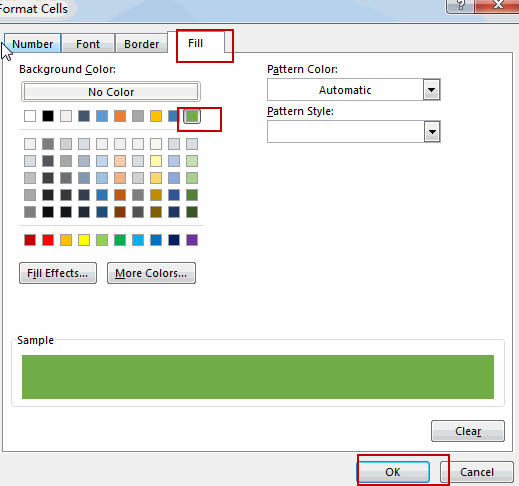#5 click Ok button. You should see that the entire rows which contain the current day have been highlighted.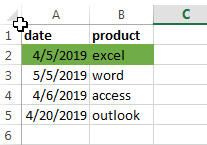### Related Functions

• Excel WEEKDAY function
The Excel WEEKDAY function returns a integer value representing the day fo the week for a given Excel date and the value is range from 1 to 7.The syntax of the WEEKDAY function is as below:=WEEKDAY (serial_number,[return_type])…
• Excel TODAY function
The Excel TODAY function returns the serial number of the current date. So you can get the current system date from the TODAY function. The syntax of the TODAY function is as below:=TODAY()…
• Excel Text function
The Excel TEXT function converts a numeric value into text string with a specified format. The TEXT function is a build-in function in Microsoft Excel and it is categorized as a Text Function. The syntax of the TEXT function is as below: = TEXT (value, Format code)…

## How to Calculate Number of Weekends between Two Dates in Excel

This post will guide you how to count the number of weekends between two given dates in Excel. How to find out how many weekends are between two dates in Excel. How to count the number of Sundays between two dates with a formula in Excel.

## Calculate the Number of Weekdays between Two Dates

Assuming that you have a list of data in range A1:B4, in which contain start dates and end dates. And you want to calculate the number of weekend days between start date and end date. How to do it. You can use a formula based on the NETWORKDAYS function and the DAYs function to calculate the number of weekends between two given dates.

Here is the formula that it will count the total number of weekend days between two given dates:

`=DAYS(B2,A2)+1-NETWORKDAYS(A2,B2)`

Type this formula into cell C2, and press Enter key on your keyboard, and then copy this formula from cell C2 to range C3:C4 to apply this formula.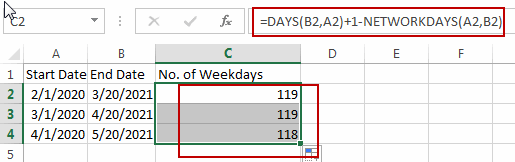Let’s see how this formula works:

The NETWORKDAYS function try to calculate the number of working days (weekdays) between two dates, and the DAYS function will count the number of days between two dates. So you can use days number to subtract working days, then you will get the number of weekends days.

## Calculate the Number of Sundays between Two Dates

If you only want to count the number of Sundays between two given dates in Excel, you can use an Excel formula based on the INT function and the WEEKDAY function. Like this:

`=INT((WEEKDAY(B1- 1)-A2+B2)/7)`

Type this formula into cell D2, and press Enter key on your keyboard.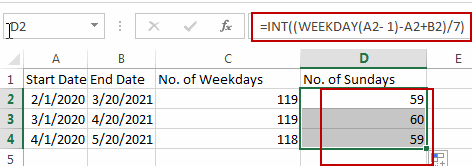Note: if you want to count the number of other week numbers (1-7, 1 is Sunday, 2 is Monday, 3 is Tuesday, 4 is Wednesday, 5 is Thursday, 6 is Friday, 7 is Saturday ) between two dates, such as: Saturday, you just need to replace the number 1 with 7 in the above formula. Like this:

`=INT((WEEKDAY(B1- 7)-A2+B2)/7)`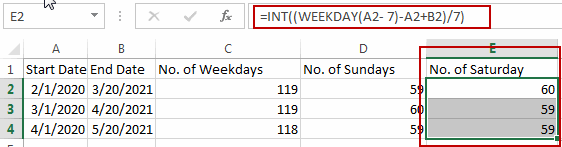### Related Functions

• Excel INT function
The Excel INT function returns the integer portion of a given number. And it will rounds a given number down to the nearest integer.The syntax of the INT function is as below:= INT (number)…
• Excel WEEKDAY function
The Excel WEEKDAY function returns a integer value representing the day fo the week for a given Excel date and the value is range from 1 to 7.The syntax of the WEEKDAY function is as below:=WEEKDAY (serial_number,[return_type])…
• Excel DAYS function
The Excel DAYS function returns the number of days between two dates.The syntax of the DAYS function is as below:= DAYS (end_date,start_date)…

## Filter Out Weekends and Weekday

This post will guide you how to filter out weekend or weekday from a list of data in Excel. How do I filter out the data in all Weekends in Excel. How to filter out all data in all weekdays in Excel.

## Filter Out data in Weekdays and Weekends

If you want to filter out the data that belong to all the weekends or weekdays in Excel, how to achieve it.  You can use a formula based on the WEEKDAY function to return a number 1-7 for each day of the week.  The number 1 indicates that it is Sunday, and the number 7 indicates that it is Saturday.  Then you can use the Filter feature to filter out the data based on the week number. Just do the following steps:

#1 Type the following formula in a cell adjacent to the date column.

`=WEEKDAY(B2)`

#2 press Enter key in your keyboard, and then drag the AutoFill Handle to other cells to apply this formula.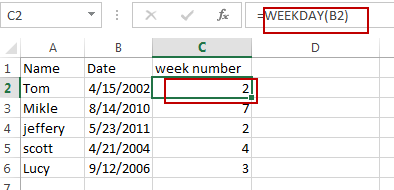#3 keeping the week numbers are selected, and go to DATA tab, click Filter command under Sort & Filter group.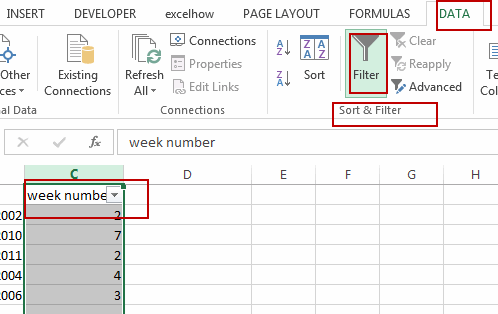#4 click Arrow button in week number filed, checked 1 and 7 to filter out all weekends. Or checked 2-6 to filter out all weekdays. Click OK button.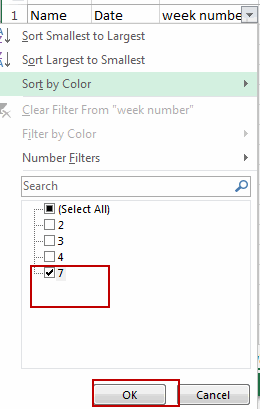#5 let’s see the result.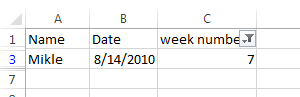### Related Functions

• Excel WEEKDAY function
The Excel WEEKDAY function returns a integer value representing the day fo the week for a given Excel date and the value is range from 1 to 7.The syntax of the WEEKDAY function is as below:=WEEKDAY (serial_number,[return_type])…

## Determine If a Date Falls on the Weekend

This post will guide you how to determine if a date falls on the weekend in Excel. How do I check if a date falls on the weekend with a formula in Excel. How to check if a date is a weekend in Excel.

## Check if a Date Falls on the Weekend

Assuming that you have a list of data that contain dates in range A1:A5, and you want to check each date if it falls on the weekend. How to achieve it. You can create a formula based on the IF function, the OR function and the WEEKDAY function. Just like this:

`=IF(OR(WEEKDAY(A1)=1,WEEKDAY(A1)=7),TRUE,FALSE)`

Type this formula into Cell B1, and press Enter key in your keyboard, and then drag the AutoFill Handle from Cell B1 to B5.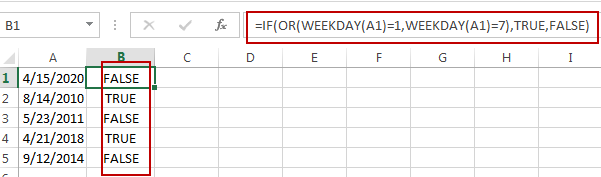This formula will check if a date falls on the weekend, it will return TRUE, otherwise, it will return FALSE.

### Related Functions

• Excel IF function
The Excel IF function perform a logical test to return one value if the condition is TRUE and return another value if the condition is FALSE. The IF function is a build-in function in Microsoft Excel and it is categorized as a Logical Function.The syntax of the IF function is as below:= IF (condition, [true_value], [false_value])….
• Excel WEEKDAY function
The Excel WEEKDAY function returns a integer value representing the day fo the week for a given Excel date and the value is range from 1 to 7.The syntax of the WEEKDAY function is as below:=WEEKDAY (serial_number,[return_type])…
• Excel OR Function
The Excel OR function used to test multiple conditions and returns TRUE if any of the conditions are TRUE.The syntax of the OR function is as below:=OR(logical1, [logical2], …)…

## Count the Number of Weekends between Two Date

This post will guide you how to count the number of weekends between two dates in Excel. How do I get the number of weekends between 2 dates with a formula in Excel. How to count weekend days between dates in Excel.

## Count the Number of Weekends between Two dates

If you want to calculate the number of weekends between two dates, you need to create a formula based on the SUM function, the INT function and the WEEKDAY function. For example, you have two dates in Cell B2:C2, and the start date is in Cell B2, and the end date is in Cell C2. You can use the following formula to achieve the result.

`=SUM(INT((WEEKDAY(B2-{1,7})+C2-B2)/7))`

Type this formula into a blank cell and then press Enter key in your keyboard.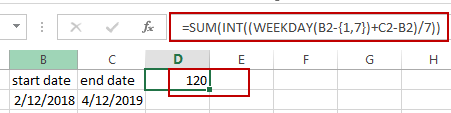The number of Weekends between those two dates is calculated.

Or you can use another formula to achieve the same result. Type the following formula into a blank cell and press Enter key.

`=C2-B2+1-NETWORKDAYS(B2,C2)`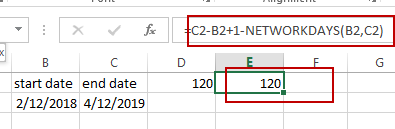### Related Functions

• Excel SUM function
The Excel SUM function will adds all numbers in a range of cells and returns the sum of these values. You can add individual values, cell references or ranges in excel.The syntax of the SUM function is as below:= SUM(number1,[number2],…)…
• Excel INT function
The Excel INT function returns the integer portion of a given number. And it will rounds a given number down to the nearest integer.The syntax of the INT function is as below:= INT (number)…
• Excel WEEKDAY function
The Excel WEEKDAY function returns a integer value representing the day fo the week for a given Excel date and the value is range from 1 to 7.The syntax of the WEEKDAY function is as below:=WEEKDAY (serial_number,[return_type])…

## Converting Week Number to Date

This post will guide you how to convert a week number to a date with a formula in Excel. How do I calculate a date from a week number and a year with formula in Excel. Or how to get week number from a given date with formula in Excel.

## Convert Week Number to Date

Assuming that you have a week number and a year number in your worksheet, and you want to get the start date and end date in that give week in that year. How to achieve it.

You need to create a formula based on the MAX function, the DATE function and the WEEKDAY function to convert week number to a date in Excel. Just do the following steps:

#1 Type the year number in Cell C1, and type the week number in Cell C2.

#2 Type this formula into the formula box of the Cell C3, then press Enter key in your keyboard. The Serial number of the start date is calculated in Cell C3.

=MAX(DATE(C1,1,1),DATE(C1,1,1)-WEEKDAY(DATE(C1,1,1),2)+(C2-1)*7+1)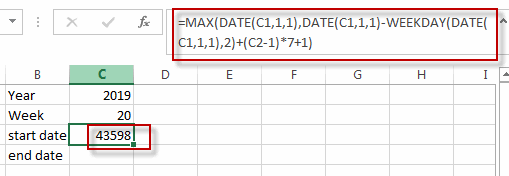#3 Type the following formula into the formula box of the Cell C4, and then press Enter key in your keyboard. And the serial number of the end date is calculated in Cell C4.

=MIN(DATE(C1+1,1,0),DATE(C1,1,1)-WEEKDAY(DATE(C1,1,1),2)+C2*7)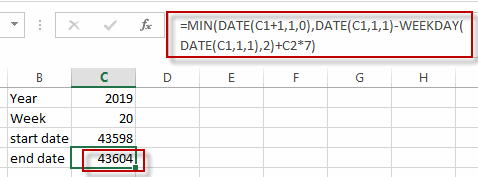#4 select the cells C3:C4 and then go to HOME tab, click Number format list box, and select Short Date item. The serial number are changed to a standard date format.  Or you can also select Long Date format from the drop-down list of the Number format.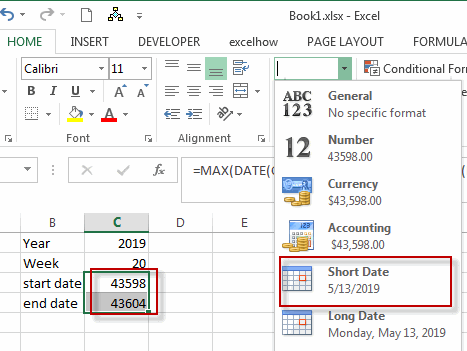## Get Week Number From a Date

If you want to get the week number from a given date, then you can use the WEEKNUM function to quickly achieve it. Just type the formula into the Cell D3:

`=WEEKNUM(C3,1)`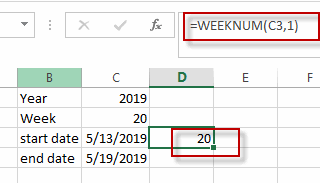### Related Functions

• Excel MIN function
The Excel MIN function returns the smallest numeric value from the numbers that you provided. Or returns the smallest value in the array.The MIN function is a build-in function in Microsoft Excel and it is categorized as a Statistical Function.The syntax of the MIN function is as below:= MIN(num1,[num2,…numn])….
• Excel MAX function
The Excel MAX function returns the largest numeric value from the numbers that you provided. Or returns the largest value in the array.= MAX(num1,[num2,…numn])…
• Excel DATE function
The Excel DATE function returns the serial number for a date.The syntax of the DATE function is as below:= DATE (year, month, day)…
• Excel WEEKDAY function
The Excel WEEKDAY function returns a integer value representing the day fo the week for a given Excel date and the value is range from 1 to 7.The syntax of the WEEKDAY function is as below:=WEEKDAY (serial_number,[return_type])…
• Excel WEEKNUM function
The Excel WEEKNUM function returns the week number of a specific date, and the returned value is ranging from 1 to 53.The syntax of the WEEKNUM function is as below:=WEEKNUM (serial_number,[return_type])…

## Get the First Monday of a Given Year

This post will guide you how to get the first Monday of a given year or date in excel. How do I calculate the first Monday of a specific year in excel. How to determine the first Monday in a given year date with a formula in excel. How to find and get the date of the first Monday of a given year date in excel.

## Get the First Monday of a Given Year

To calculate the first Monday of the year or given any date, you can create a new complex formula based on the DATE function, the YEAR function and the WEEKDAY function.

Assuming that you have a date list in the range of cells B1:B4 in your current worksheet, and if you want to get the first Monday for the year date in each cell, and you can write down one formula as below:

`=DATE(YEAR(B1),1,8)-WEEKDAY(DATE(YEAR(B1),1,6))`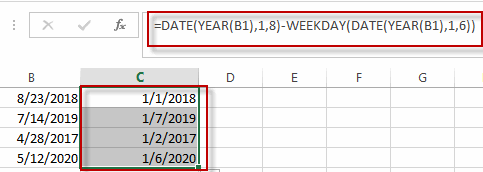Type this formula into the formula box of a blank cell such as: C1, then press Enter key in your keyboard, and drag the AutoFill Handler over other cells to apply this formula.

You can also use another formula based on the DATE function, the CHOOSE function and the WEEKDAY function to create the same result. Like this:

`=DATE(B1,1,1)+CHOOSE(WEEKDAY(DATE(B1,1,1),2),0,6,5,4,3,2,1)`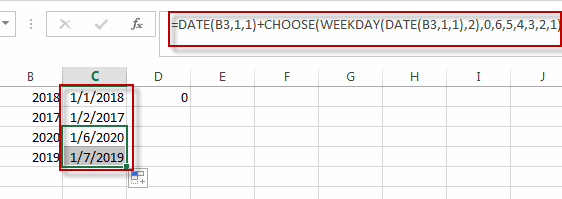### Related Functions

• Excel WEEKDAY function
The Excel WEEKDAY function returns a integer value representing the day fo the week for a given Excel date and the value is range from 1 to 7.The syntax of the WEEKDAY function is as below:=WEEKDAY (serial_number,[return_type])…
• Excel DATE function
The Excel DATE function returns the serial number for a date.The syntax of the DATE function is as below:= DATE (year, month, day)…
• Excel YEAR function
The Excel YEAR function returns a four-digit year from a given date value, the year is returned as an integer ranging from 1900 to 9999. The syntax of the YEAR function is as below:=YEAR (serial_number)…
• Excel Choose Function
The Excel CHOOSE function returns a value from a list of values. The CHOOSE function is a build-in function in Microsoft Excel and it is categorized as a Lookup and Reference Function.The syntax of the CHOOSE function is as below:=CHOOSE (index_num, value1,[value2],…)…

## Excel WEEKDAY Function

This post will guide you how to use Excel WEEKDAY function with syntax and examples in Microsoft excel.

### Description

The Excel WEEKDAY function returns a integer value representing the day fo the week for a given Excel date and the value is range from 1 to 7. so you can use this function to get the weekday number for a supplied date. and it will returns 1 for Sunday and returns 7 for Saturday. you also can use the WEEKDAY function to combine with other formulas or functions.

The WEEKDAY function is a build-in function in Microsoft Excel and it is categorized as a DATE and TIME Function.

The WEEKDAY function is available in Excel 2016, Excel 2013, Excel 2010, Excel 2007, Excel 2003, Excel XP, Excel 2000, Excel 2011 for Mac.

### Syntax

The syntax of the WEEKDAY function is as below:

`=WEEKDAY (serial_number,[return_type])`

Where the WEEKDAY function arguments is:

• Serial_number –This is a required argument. An Excel date that you want to get the weekday of. the dates should be typed by the DATE function to make sure it is a valid Excel date. For example, use DATE(2018,3,20) to pass into the WEEKDAY function and then it will return the weekday of this date.
• return_type – This is an optional argument. A number that determines the type of return value.
 Return_type Number returned 1 or omitted Numbers 1 (Sunday) through 7 (Saturday). Behaves like previous versions of Microsoft Excel. 2 Numbers 1 (Monday) through 7 (Sunday). 3 Numbers 0 (Monday) through 6 (Sunday). 11 Numbers 1 (Monday) through 7 (Sunday). 12 Numbers 1 (Tuesday) through 7 (Monday). 13 Numbers 1 (Wednesday) through 7 (Tuesday). 14 Numbers 1 (Thursday) through 7 (Wednesday). 15 Numbers 1 (Friday) through 7 (Thursday). 16 Numbers 1 (Saturday) through 7 (Friday). 17 Numbers 1 (Sunday) through 7 (Saturday).

Note:

• If serial_number is out of range for the current date base value, a #NUM! error is returned.
• If return_type is out of the range specified in the table above, a #NUM! error is returned.
• A serial date is how the Microsoft Excel stores dates and it represents the number of days since 1900-01-01, so the January 1, 1900 date is serial number 1 by default.

### Excel WEEKDAY Function Examples

The below examples will show you how to use Excel WEEKDAY Function to return a weekday of a date.

1# get day of the week with the default return type 1, enter the following formula in Cell B1.

=WEEKDAY(DATE(2018,3,20))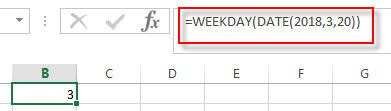it means that the weekday of that date is Tuesday.

### Related Functions

• Excel WEEKNUM function
The Excel WEEKNUM function returns the week number of a specific date, and the returned value is ranging from 1 to 53.The syntax of the WEEKNUM function is as below:=WEEKNUM (serial_number,[return_type])…
• Excel DAY function
The Excel DAY function returns a day of a date (from 1 to 31).The DAY function is a build-in function in Microsoft Excel and it is categorized as a DATE and TIME Function.The syntax of the DAY function is as below:= DAY (date_value)…
• Excel EDATE function
TThe Excel EDATE function returns the serial number that represents the date that is a specified number of months before or after a specified date.The syntax of the EDATE function is as below:=EDATE (start_date, months)…
• Excel DATE function
The Excel DATE function returns the serial number for a date.The syntax of the DATE function is as below:= DATE (year, month, day)…

### More Excel WEEKDAY Function Examples

• Calculate Thanksgiving Date
You can use a simple arithmetic to calculate the possible date range for the US Thanksgiving, it falls on the fourth Thursday in November. And you can create a formula based on the DATE function and the WEEKDAY function.…
• Convert Date to Day of Week in Excel (get day name from date )
If you want to get the day name from a date in excel, you can use the TEXT function with a specified format code like “ddd” or “dddd”..…
• Get the First Monday of a Given Year
To calculate the first Monday of the year or given any date, you can create a new complex formula based on the DATE function, the YEAR function and the WEEKDAY function……
• Converting Week Number to Date
You need to create a formula based on the MAX function, the DATE function and the WEEKDAY function to convert week number to a date in Excel.…

## Convert Date to Day of Week in Excel (get day name from date )

In excel, how to get the day of week from an excel date value. How to return a day name of the week from a date that is contained in a cell in Microsoft Excel. This post will guide you how to convert date to day of week only using Text Function in Microsoft Excel.

## Convert date to day of week with Text Function (get day of week from date)

If you want to get the day name from a date in excel, you can use the TEXT function with a specified format code like “ddd” or “dddd”. We can try to use the below TEXT formula in excel:

```=TEXT(date,"ddd")
=TEXT(date, "dddd")```

If you want to get the day name of the week as an abbreviation from a date, such as: Mon, Tue, etc. Enter the following TEXT formula in the Cell C1 that you want to get the day name of the week, and then press ENTER:

`=TEXT(B1,"ddd")`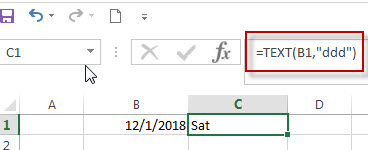If you want to get the full day name of the week, such as: Monday, Tuesday, etc. Enter the following TEXT formula in the Cell C1 that you want to get the day name of the week, then press ENTER:

`=TEXT(B1,"dddd")`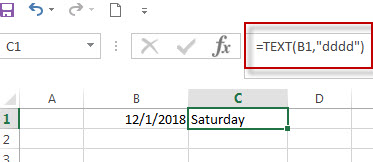## Get a Day Name of the Week Using WEEKDAY and CHOOSE function

The WEEKDAY function will get a day number of the week form a date.

We can use the returned value of WEEKDAY function as the first argument within the CHOOSE function. The CHOOSE function will use the returned weekday number as the first argument to return the nth day name from a list of day name.

So we can use the following CHOOSE function in combination with WEEKDAY function to get the full day name of the week from a date.

`=CHOOSE(WEEKDAY(B1),"Sunday","Monday","Tuesday","Wedesday","Thusday","Friday","Saturday")`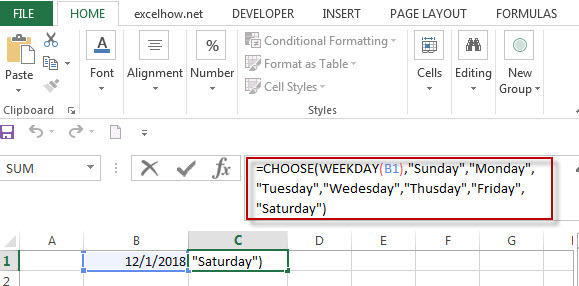For more format codes in excel formatting, you can refer to the below table:

 Format Code Description Examples 0 only display digits in its place #.00 – Forces the function to display two decimal places =Text(34.234,”\$##.00″) result: \$34.23 # Display the placeholder =Text(4.527,”#.##) result: 4.53 . the position of Decimal Point =Text(342.2,”0.00″) result: 342.20 d Day of the month or day of week d- one or two digit number (1-31) dd- two digit number (01-31) ddd-abbreviated day of week (Mon to Sun) dddd-full name of day of week(Monday to Sunnday) =Text(TODAY(),”DDDD”) result: Monday m The Month of the Year m- one or two digit number mm-two digit number mmm-abbreviated month(Jan to Dec) mmmm-full name of month(January to December)) =Text(TODAY(),”MM/DD/YY”) result:11/06/17 y year yy-two digit representation of year(e.g.01,17) yyyy-four digit representation of year(e.g. 2001,2017) =Text(TODAY(),”MM/DD/YY”) result:11/06/17

### Related Formulas

• Excel Convert numbers to Text
The Text function will accept a numeric value as the first argument, then based on the format code in the second argument to convert the number to text. You can convert all the standard number formats such as: dates, times, currency to Text string in excel.…
• Convert date to month name with Text Function
If you want to convert the date to a month name, you can use the TEXT function with a specified format code like “mmm”in excel.  You can try to run the following TEXT formula:=TEXT(B1,”mmm”)
• Convert Date to text with Text Function in Excel
you can use TEXT function in excel to convert dates to text in a specific format code. For example, The TEXT function can use the following patterns, like as: “mm/dd/yyyy”, “yyyy/mm/dd”, etc.
• Convert date to month and year only in excel
If you want to convert the date to month and year only, you can use “yyyymm” format code within the TEXT function in excel, so you can write down the below TEXT formula:=TEXT(date,”yyyymm”)
• Convert date to month and day only in excel
If you want to convert the date (mm/dd/yyyy) to month and day only, you can use “mm dd” format code within the TEXT function in excel, so you can write down the below TEXT formula: =TEXT(date,”mm dd”)

### Related Functions

• Excel Text function
The Excel TEXT function converts a numeric value into text string with a specified format. The TEXT function is a build-in function in Microsoft Excel and it is categorized as a Text Function. The syntax of the TEXT function is as below: = TEXT (value, Format code)…
• Choose function in excel
The Excel CHOOSE function returns a value from a list of values. The CHOOSE function is a build-in function in Microsoft Excel and it is categorized as a Lookup and Reference Function.The syntax of the CHOOSE function is as below:=CHOOSE (index_num, value1,[value2],…)…
• Excel WEEKDAY function
The Excel WEEKDAY function returns a integer value representing the day fo the week for a given Excel date and the value is range from 1 to 7.The syntax of the WEEKDAY function is as below:=WEEKDAY (serial_number,[return_type])…